# ML Aggarwal Class 7 Solutions for ICSE Maths Chapter 16 Perimeter and Area Objective Type Questions

## ML Aggarwal Class 7 Solutions for ICSE Maths Chapter 16 Perimeter and Area Objective Type Questions

Mental Maths

Class 7 Maths Chapter 16 Perimeter and Area Question 1. Fill in the blanks:
(i) The perimeter of a regular polygon = ……….. × length of a side.
(ii) The unit of measurement of the area is ……….
(iii) The perimeter of a rhombus is = 4 × ………
(iv) An area of 1 km2 = ……… hectare
(v) If the perimeter of a parallelogram is 40 cm and the length of one side is 12 cm, then the length of the adjacent side is ……
(vi) To find the cost of polishing a table-top, we need to find the ………. of the table-top.
(vii) The ratio of circumference to the diameter of a circle is ………..
(viii) If the area of a triangular piece of cardboard is 90 cm2, then the length of the altitude corresponding to 20 cm long base is ………. cm.
Solution: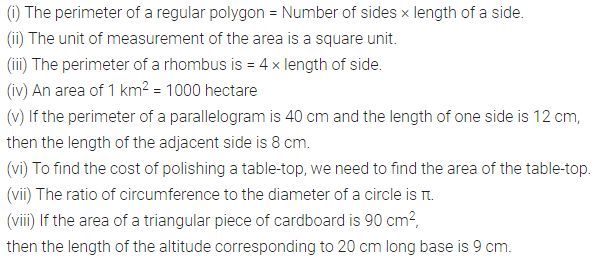ML Aggarwal Class 7 Solutions Chapter 16 Question 2. State whether the following statements are true (T) or false (F):
(i) A diagonal of a rectangle divides it into two right-angled triangles of equal areas.
(ii) A diagonal of a parallelogram divides it into two triangles of equal areas.
(iii) If the perimeter of two parallelograms is equal, then their areas are also equal.
(iv) All parallelogram having equal areas have the same perimeters.
(v) The area of a circle of diameter d is πd2.
(vi) Area of a parallelogram = product of lengths of its two adjacent sides.
Solution:Multiple Choice Questions

Choose the correct answer from the given four options (3 to 14):
Question 3.
If the perimeter of a square is 24 cm, then its area is
(a) 16 cm2
(b) 24 cm2
(c) 36 cm2
(d) 36 m2
Solution: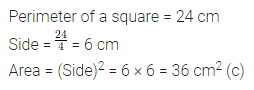ML Aggarwal Class 7 Chapter 16 Question 4. If the area of a parallelogram is 54 cm2 and the length of one side is 7.5 cm, then the corresponding height is
(a) 7.2 cm
(b) 14.4 cm
(c) 3.6 cm
(d) 13.5 cm
Solution:Perimeter and Area Class 7 Question 5.
If the base of a triangle is doubled and its height is halved, then the area of the resulting triangle
(a) decreases
(b) increases
(c) doubles
(d) remains the same
Solution: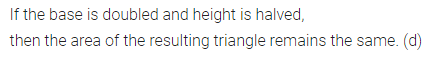ML Aggarwal Class 7 Question 6. If the height of a parallelogram is doubled and base tripled, then its area becomes
(a) 2 times
(b) 3 times
(c) 6 times
(d) 12 times
Solution:Perimeter and Area Class 7 Question 7. The circumference of the circle with diameter 28 cm is
(a) 44 cm
(b) 88 cm
(c) 176 cm
(d) 616 cm
Solution:ML Aggarwal Class 7 Perimeter and Area Question 8. The ratio of circumference to the area of a circle of radius r units is
(a) 2 : r
(b) r : 2
(c) 1 : r
(d) π : r
Solution:ML Aggarwal Class 9 Solutions ICSE Chapter 16 Question 9. If the area of a circle is numerically equal to its circumference, then the radius of the circle is
(a) 1 unit
(b) 2 units
(c) 3 units
(d) 4 units
Solution:Question 10.
The area of a circle of diameter d is
(a) 2πd2
(b) πd2
(c) $$\frac { \pi { d }^{ 2 } }{ 2 }$$
(d) $$\frac { \pi { d }^{ 2 } }{ 4 }$$
Solution:ML Aggarwal Maths for Class 7 Solutions Pdf Question 11. If the ratio of the radii of two circles is 2 then the ratio of their circumferences is
(a) 2 : 3
(b) 3 : 2
(c) 4 : 9
(d) 9 : 4
Solution:Question 12.
If the ratio of the radii of two circles is 3 : 5, then the ratio of their areas is
(a) 3 : 5
(b) 5 : 3
(c) 25 : 9
(d) 9 : 25
Solution:Question 13.
The perimeter of a semicircle (including its diameter) of radius 7 cm is
(a) 22 cm
(b) 29 cm
(c) 36 cm
(d) 44 cm
Solution:Question 14.
Area of a rectangle and the area of a circle are equal. If the dimensions of the rectangle are 14 cm × 11 cm, then the radius of the circle is
(a) 21 cm
(b) 14 cm
(c) 10.5 cm
(d) 7 cm
Solution: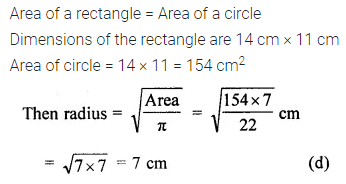Higher Order Thinking Skills (HOTS)

Question 1.
In the given figure, ABDE is a parallelogram, find the area of the trapezium ACDE.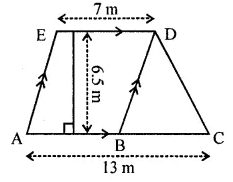Solution: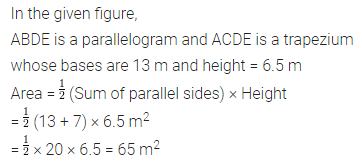Question 2.
In the given figure, the length of the rectangle is 28 cm. Find the area of the shaded region.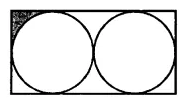Solution:Question 3.
In the given figure, ABCD is a square of side 14 cm. A, B, C, and D are centers of circular arcs of equal radius. Find the perimeter and the area of the shaded region.Solution: